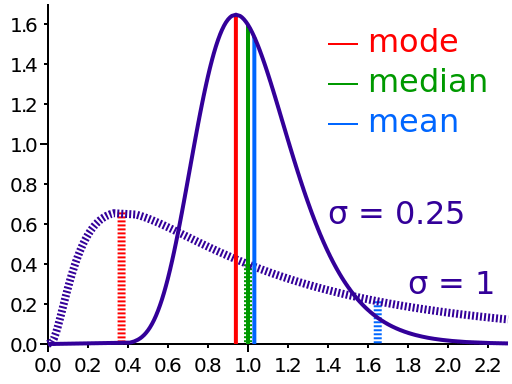# How to Emulate the MEDIAN() Aggregate Function Using Inverse Distribution Functions

Some databases are awesome enough to implement the `MEDIAN()` aggregate function. Remember that the `MEDIAN()` is sligthly different from (and often more useful than) the `MEAN()` or `AVG()` (average). While the average is calculated as the `SUM(exp) / COUNT(exp)`, the `MEDIAN()` tells you that 50% of all values in the sample are higher than the `MEDIAN()` whereas the other 50% of the set are lower than the `MEDIAN()`. So, in other words, if you take the following query:
```
WITH t(value) AS (
SELECT 1   FROM DUAL UNION ALL
SELECT 2   FROM DUAL UNION ALL
SELECT 3   FROM DUAL
)
SELECT
avg(value),
median(value)
FROM
t;

```
… then both average and median are the same:
```avg   median
2     2
```
But if you heavily skew your data like this:
```
WITH t(value) AS (
SELECT 1   FROM DUAL UNION ALL
SELECT 2   FROM DUAL UNION ALL
SELECT 100 FROM DUAL
)
SELECT
avg(value),
median(value)
FROM
t;

```
Then your average will also be skewed, whereas your median will still indicate where most of the values are in your sample
```avg      median
34.333   2
```
The above sample is of course statistically insignificant, but you can easily see that the effect can be dramatic and relevant, if you have more data:Image license CC-BY-SA 3.0. Uploaded to Wikipedia by Cmglee The skewing effect is very important in statistics and in order to make any interesting claim about anything, using percentiles is most often more useful than using averages. Take the average income vs. the median income in a country, for instance. While the average income in the U.S. (and in many other countries) has been steadily increasing, the median income has seen a decline over the past decade. This is due to wealth being heavily skewed towards the super-rich more and more. This blog is not about politics but about Java and SQL, so let’s get back into calculating the actual facts.

## Using precentiles in SQL

As we’ve seen before, the `MEDIAN()` divides a sample into two equally-sized groups and takes the value “between” those two groups. This particular value is also called the 50th percentile because 50% of all values in the sample are lower than the `MEDIAN()`. We can thus establish:
• `MIN(exp)`: The 0-percentile
• `MEDIAN(exp)`: The 50th-percentile
• `MAX(exp)`: The 100th-percentile
All of the above are special cases of percentiles, and while `MIN()` and `MAX()` are supported in all SQL databases (and the SQL standard), `MEDIAN()` is not in the SQL standard and only supported by the following jOOQ databases:
• CUBRID
• HSQLDB
• Oracle
• Sybase SQL Anywhere
There is another way of calculating the `MEDIAN()` in particular and any sort of percentile in general in the SQL standard, and since PostgreSQL 9.4 also in PostgreSQL using …

## Ordered-set aggregate functions

Interestingly, apart from window functions, you can also specify `ORDER BY` clauses to certain aggregate functions that aggregate data based on ordered sets. One such function is the SQL standard `percentile_cont` function, which takes the percentile as an argument, and then accepts an additional `WITHIN GROUP` clause that takes an `ORDER BY` clause as an argument. These particular ordered-set functions are also called inverse distribution functions, because we want to find where a particular percentile is located in the distribution of all values in the sample (if you’re not scared by the math, check out the wikipedia article) So, in PostgreSQL 9.4+, the `MEDIAN()` function can be emulated like this:
```
WITH t(value) AS (
SELECT 1   UNION ALL
SELECT 2   UNION ALL
SELECT 100
)
SELECT
avg(value),
percentile_cont(0.5) WITHIN GROUP (ORDER BY value)
FROM
t;

```
This interesting syntax is standardised and may be known to some of you from Oracle’s LISTAGG(), which allows to aggregate values into concatenated strings:
```
WITH t(value) AS (
SELECT 1   FROM DUAL UNION ALL
SELECT 2   FROM DUAL UNION ALL
SELECT 100 FROM DUAL
)
SELECT
listagg(value, ', ') WITHIN GROUP (ORDER BY value)
FROM
t;

```
This query yields simply:
```listagg
---------
1, 2, 100
```
On a side-note: `LISTAGG()` is, of course, completely useless, because it returns `VARCHAR2`, which again has a max length of 4000 in Oracle. Useless…

## Emulation out-of-the-box with jOOQ

As always, jOOQ will emulate these kinds of things out of the box. You can either use the `DSL.median()` function, or with the upcoming jOOQ 3.6, the new `DSL.percentileCont()` function to produce the same value:
```
DSL.using(configuration)
.select(
median(T.VALUE),
percentileCont(0.5).withinGroupOrderBy(T.VALUE)
)
.from(T)
.fetch();

```